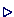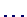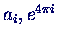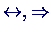## MathematicsThe features to layout complicated mathematical formulas in a very pleasing way are at the heart of LATEX and one of the reasons for its widespread use in the scientific community.The basic environments for mathematical formulas are

math
formulas appear in the running text. Short form: $$.
displaymath
produces a new paragraph. Short form: $$
equation
like displaymath, but the equations are numbered.

Inside one of these environments LATEX uses special formatting rules, the so called math mode''. Many of the following commands only work in math mode.LATEX sets formulas in a running line in a more condensed form than in a displaymath or equation environment.The following table shows some of the many special mathematical commands:

 description name result greek letters \alpha, \beta\Gamma, \Deltasub and superscripts a_i, e^{4\pi i}relations \leq, \geq, \subsetarrows \leftrightarrow, \Rightarrowfractions \frac{2}{4-x}large brackets \left(\frac{2}{4-x} \right)square roots \sqrt{42+y}integrals \int_0^1 x^2 dxAs always grouping with {, } has to be used properly. Compare the following:

 LATEX input result a_n+1a_{n+1}A mathematical input file: math.tex

The generated document looks like:Peter Junglas 15.5.2000